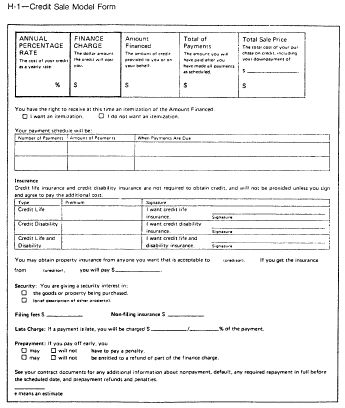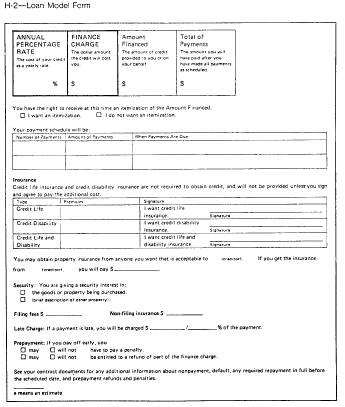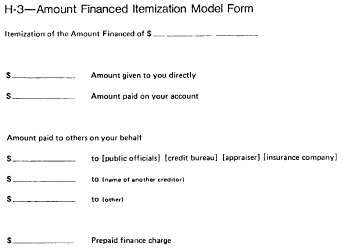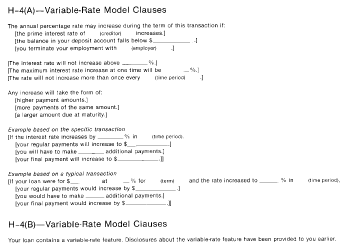# Title 12

## Appendix H to Part 226 - Closed-End Model Forms and Clauses

### 12:3.0.1.1.7.9.8.1.23 : Appendix H

Appendix H to Part 226 - Closed-End Model Forms and Clauses H-1 Credit Sale Model Form (§ 226.18) H-2 Loan Model Form (§ 226.18) H-3 Amount Financed Itemization Model Form (§ 226.18(c)) H-4(A) Variable-Rate Model Clauses (§ 226.18(f)(1)) H-4(B) Variable-Rate Model Clauses (§ 226.18(f)(2)) H-4(C) Variable-Rate Model Clauses (§ 226.19(b)) H-4(D) Variable-Rate Model Clauses (§ 226.20(c)) H-4(E) - Fixed-Rate Mortgage Interest Rate and Payment Summary Model Clause (§ 226.18(s)) H-4(F) - Adjustable-Rate Mortgage or Step-Rate Mortgage Interest Rate and Payment Summary Model Clause (§ 226.18(s)) H-4(G) - Mortgage with Negative Amortization Interest Rate and Payment Summary Model Clause (§ 226.18(s)) H-4(H) - Fixed-Rate Mortgage with Interest-Only Interest Rate and Payment Summary Model Clause (§ 226.18(s)) H-4(I) - Adjustable-Rate Mortgage Introductory Rate Disclosure Model Clause (§ 226.18(s)(2)(iii)) H-4(J) - Balloon Payment Disclosure Model Clause (§ 226.18(s)(5)) H-4(K) - No Guarantee to Refinance Statement Model Clause (§ 226.18(t)) H-5 Demand Feature Model Clauses (§ 226.18(i)) H-6 Assumption Policy Model Clause (§ 226.18(q)) H-7 Required Deposit Model Clause (§ 226.18(r)) H-8 Rescission Model Form (General) (§ 226.23) H-9 Rescission Model Form (Refinancing (with Original Creditor)) (§ 226.23) H-10 Credit Sale Sample H-11 Installment Loan Sample H-12 Refinancing Sample H-13 Mortgage with Demand Feature Sample H-14 Variable-Rate Mortgage Sample (§ 226.19(b)) H-15 Graduated-Payment Mortgage Sample H-16 Mortgage Sample H-17(A) Debt Suspension Model Clause H-17(B) Debt Suspension SampleH-4(C) - Variable-Rate Model Clauses

This disclosure describes the features of the adjustable-rate mortgage (ARM) program you are considering. Information on other ARM programs is available upon request.

How Your Interest Rate and Payment Are Determined

• Your interest rate will be based on [an index plus a margin] [a formula].

• Your payment will be based on the interest rate, loan balance, and loan term.

- [The interest rate will be based on (identification of index) plus our margin. Ask for our current interest rate and margin.] - [The interest rate will be based on (identification of formula). Ask us for our current interest rate.] - Information about the index [formula for rate adjustments] is published [can be found] ________. - [The initial interest rate is not based on the (index) (formula) used to make later adjustments. Ask us for the amount of current interest rate discounts.] How Your Interest Rate Can Change

• Your interest rate can change (frequency).

• [Your interest rate cannot increase or decrease more than ___ percentage points at each adjustment.]

• Your interest rate cannot increase [or decrease] more than ___ percentage points over the term of the loan.

• Your payment can change (frequency) based on changes in the interest rate.

• [Your payment cannot increase more than (amount or percentage) at each adjustment.]

• You will be notified in writing ____ days before the due date of a payment at a new level. This notice will contain information about your interest rates, payment amount, and loan balance.

• [You will be notified once each year during which interest rate adjustments, but no payment adjustments, have been made to your loan. This notice will contain information about your interest rates, payment amount, and loan balance.]

• [For example, on a \$10,000 [term] loan with an initial interest rate of ____ [(the rate shown in the interest rate column below for the year 19 ____)] [(in effect (month) (year)], the maximum amount that the interest rate can rise under this program is ____ percentage points, to ____%, and the monthly payment can rise from a first-year payment of \$____ to a maximum of \$____ in the _____ year. To see what your payments would be, divide your mortgage amount by \$10,000; then multiply the monthly payment by that amount. (For example, the monthly payment for a mortgage amount of \$60,000 would be: \$60,000 ÷ \$10,000 = 6; 6 × ____ = \$____ per month.)]

[Example

The example below shows how your payments would have changed under this ARM program based on actual changes in the index from 1982 to 1996. This does not necessarily indicate how your index will change in the future.

The example is based on the following assumptions:

 Amount \$10,000 Term _____ Change date _____ Payment adjustment (frequency) Interest adjustment (frequency) [Margin] * ____ Caps ____ [periodic interest rate cap] ____ [lifetime interest rate cap ____ [payment cap] [Interest rate carryover] [Negative amortization] [Interest rate discount] ** Index.......(identification of index or formula)